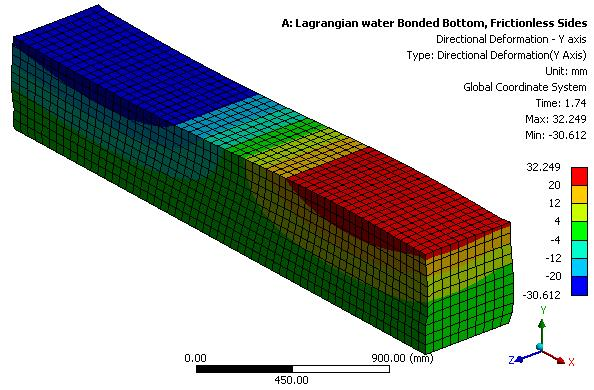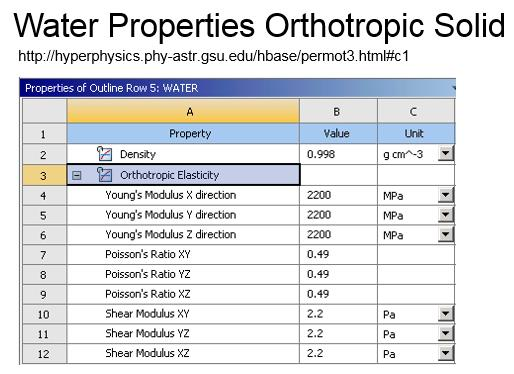## General Mechanical

•niklas37
Subscriber

Hi all,

how can I create a seismic analysis of a water filled tank using Ansys? Which type of analysis could use for this problem and how can I couple the analysis the right way?

Thanks

•pgl
Ansys Employee

This depends on how important the water sloshing is to your application: If the tank is completely full, you could assume the water is a solid. That might be an oversimplification. You could also assume that the tank is a rigid body. For best results you'll need to perform a two way fluid-structural interaction simulation. That will capture the movement of the fluid and the deformation of the tank.There's quite a few published tutorials example on YouTube, for example, here's an AIM FSI tutorial to get you started:

Also, here's two videos of a seismic simulation of fluid filled tanks:

•Raef.Kobeissi
Subscriber

Hello niklas37, I've done a similar simulation before, this is the tutorial for it, have a look and please let me know if it answers your question!

Cheers

•peteroznewman
Subscriber

Here is how to build a model of sloshing in a tank using Explicit Dynamics. It may not be better than Fluent, but it seemed like a simple model to build.

Start with a surface model of the tank. I drew a 1x3x1 m rectangular five sided box. Create a solid body to represent the water in the tank. I drew a 1x3x0.5 m rectangular solid. In DesignModeler, change the water body from Solid to Fluid. I then used symmetry to model one half. Let's try to model the case where the sloshing is caused by the rectangular tank having a sinusoidal motion along the length of the tank. In Workbench, drop an Explicit Dynamics block on the Project Schematic. Apply the material properties to the tank and the water. Add a gravity load. Add supports. In this example, hold three edges, and paste tabular input from a spreadsheet of the time, x, y and z displacements. I created a sine wave function that has an amplitude of 20 mm and a period of 2 seconds. I edited the default contact from bonded to frictionless on the sides of the tank. Configure Analysis Settings to set the end time and the report time. Solve. After 1 hour of compute time to get to 2 seconds, I plotted the body of water at the 1.74 second mark. Watching the animation is better.Here is how to build a tank model in Fluent. Looks like a lot more steps to me.

•peteroznewman
Subscriber

pgl mentioned in his post above that water can be modeled as a solid if the tank is full.

I used that method in a model built to study the stress in a plastic tube filled with water when it hits the floor from a 15 inch (38 cm) drop height. Though many people think of Explicit Dynamics when a drop test simulation is needed, I didn't have a license at that time, but I had a Transient Dynamics license so I used the implicit solver to run the model and the results were excellent.

Here are the water properties I used.See this discussion for more information on this model.

•kashfia.mahin
Subscriber
nCan you share the file here?.